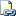# DATETIME2FROMPARTS (Transact-SQL)

Returns a datetime2 value for the specified date and time and with the specified precision.Transact-SQL Syntax Conventions

## Syntax

``````DATETIME2FROMPARTS ( year, month, day, hour, minute, seconds, fractions, precision )
``````

## Arguments

• year
Integer expression specifying a year.

• month
Integer expression specifying a month.

• day
Integer expression specifying a day.

• hour
Integer expression specifying hours.

• minute
Integer expression specifying minutes.

• seconds
Integer expression specifying seconds.

• fractions
Integer expression specifying fractions.

• precision
Integer literal specifying the precision of the datetime2 value to be returned.

## Return Types

datetime2 ( precision )

## Remarks

DATETIME2FROMPARTS returns a fully initialized datetime2 value. If the arguments are not valid, an error is raised. If required arguments are null, then null is returned. However, if the precision argument is null, then an error is raised.

The fractions argument depends on the precision argument. For example, if precision is 7, then each fraction represents 100 nanoseconds; if precision is 3, then each fraction represents a millisecond. If the value of precision is zero, then the value of fractions must also be zero; otherwise, an error is raised.

This function is capable of being remoted to SQL Server 2012 servers and above. It will not be remoted to servers that have a version below SQL Server 2012.

## Examples

### A. Simple example without fractions of a second

``````SELECT DATETIME2FROMPARTS ( 2010, 12, 31, 23, 59, 59, 0, 0 ) AS Result;
``````

Here is the result set.

``````Result
---------------------------
2010-12-31 23:59:59.0000000

(1 row(s) affected)
``````

### B. Example with fractions of a second

The following example demonstrates the use of the fractions and precision parameters:

1. When fractions has a value of 5 and precision has a value of 1, then the value of fractions represents 5/10 of a second.

2. When fractions has a value of 50 and precision has a value of 2, then the value of fractions represents 50/100 of a second.

3. When fractions has a value of 500 and precision has a value of 3, then the value of fractions represents 500/1000 of a second.

``````SELECT DATETIME2FROMPARTS ( 2011, 8, 15, 14, 23, 44, 5, 1 );
SELECT DATETIME2FROMPARTS ( 2011, 8, 15, 14, 23, 44, 50, 2 );
SELECT DATETIME2FROMPARTS ( 2011, 8, 15, 14, 23, 44, 500, 3 );
GO
``````

Here is the result set.

``````----------------------
2011-08-15 14:23:44.5

(1 row(s) affected)

----------------------
2011-08-15 14:23:44.50

(1 row(s) affected)

----------------------
2011-08-15 14:23:44.500

(1 row(s) affected)
``````